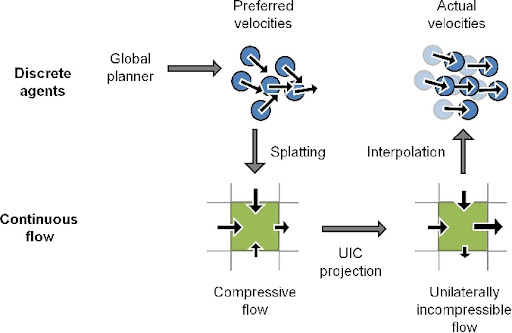## Thursday, 21 January 2010

### Paper: Aggregate Dynamics for Dense Crowd Simulation (2009)

Ming Lin's latest work has been featured in the recent publication of SIGGRAPH Asia 2009. The author presents an innovative method that is able to simulate tens of thousands of agents at interative rates.

The work achieves these rates by combining a local behavioural model with a global planner such as Continuum Crowds or Adaptive elastic Roadmaps (AeRO).Image taken from gamma.cs.unc.edu
The image above shows an overview of the algorithm. So how does this actually work?

First of all, as I mentioned this approach combines a local behaviour with a global planner. The global planner here computes the flow of agents around an environment. The steps are described below:
• Each agent is given a position and a current velocity computed.
• Using the global planner such as the roadmap based method or Continuum Crowds method, a preferred velocity is then computed for each agent.
• The navigable area in the environment is divided into a discrete grid of cells. The flow of particle for each cell is computed, using the current velocity and density of agents in the cell.
• For each agent, a new velocity is computed, by interpolating the flow velocity with the desired velocity weighted by the local density at each cell.
• Velocities of agents are constrained in dense areas by the global particle flow. In coarser areas, the agent's velocity are influence less by the global flow, and more by the velocity for the cell.
• Finally, collision detection is applied, as well as a minimum distance for each pair of individual agents, similar to the continuum crowds simulation.
Here's a video of the simulation:

Aggregate Dynamics for Dense Crowd Simulation

A good hybrid approach used here that decreases the computation time considerably to accomodate a very large number of agents at interactive rates. The Unilaterally Incompressibility Constraint (UIC) introduced here that allows the interpolation between the global and local approach is something that has interested me.